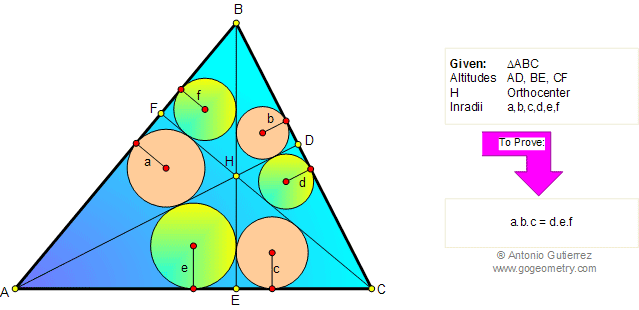Home Geometry Problems 71-80 Altitude Circle Tangent Line View or post a solution     by Antonio Gutierrez
 Problem 79. Triangle Similarity, Altitudes, Orthocenter, Incircles, Inradii. Level: High School, College, SAT Prep. Given a triangle ABC, the altitudes AD, BE, and CF, the orthocenter H. If a,b,c,d,e, and f are the inradii of triangles AFH, BDH, CEH, CDH, AEH, and BFH respectively, prove that a.b.c = d.e.f.HINTS: Triangle Proposition: The sum of the measures of the three angles of a triangle is 180.Altitude is the perpendicular line segment from one vertex to the line that contains the opposite side.Similar Triangles are triangles whose corresponding angles are congruent and whose corresponding sides are in proportion. Proposition. Triangle Similarity AA. If two angles of one triangle are congruent to two angles of another triangle, the two triangles are similar.Proposition: If two triangles are similar, then the ratio of any two corresponding segments (such as altitudes, medians, inradii, or angle bisectors) equals the ratio of any two corresponding sides. Recent Additions